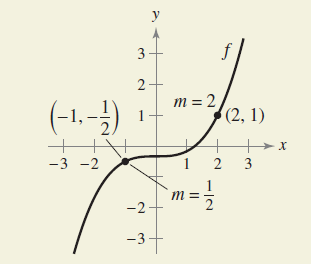×
Get Full Access to Calculus: Early Transcendental Functions - 6 Edition - Chapter 3.6 - Problem 70
Get Full Access to Calculus: Early Transcendental Functions - 6 Edition - Chapter 3.6 - Problem 70

×

# ?How Do You See It? Use the information in the graph of f below. (a) What is the slope of the tangent line to the graph of f-1 at the poISBN: 9781285774770 141

## Solution for problem 70 Chapter 3.6

Calculus: Early Transcendental Functions | 6th Edition

• Textbook Solutions
• 2901 Step-by-step solutions solved by professors and subject experts
• Get 24/7 help from StudySoup virtual teaching assistantsCalculus: Early Transcendental Functions | 6th Edition

4 5 1 285 Reviews
15
0
Problem 70

How Do You See It? Use the information in the graph of f below.(a) What is the slope of the tangent line to the graph of f-1 at the point? Explain.

(b) What is the slope of the tangent line to the graph of f-1 at the point (1, 2)? Explain.

Text Transcription:

Step-by-Step Solution:

Step 1 of 5) Just as we must be careful with a function when determining if it satisfies a property throughout its domain (like continuity or the Intermediate Value Property), so must we also be careful with a vector field in determining the properties it may or may not have over its assigned domain.

Step 2 of 2

## Discover and learn what students are asking

Calculus: Early Transcendental Functions : Riemann Sums and Definite Integrals
?In Exercises 3–8, evaluate the definite integral by the limit definition. $$\int_{-2}^{3} x d x$$

Statistics: Informed Decisions Using Data : Testing the Significance of the Least-Squares Regression Model
?Concrete. As concrete cures, it gains strength. The following data represent the 7-day and 28-day strength (in pounds per square inch) of a certain ty

Unlock Textbook Solution